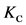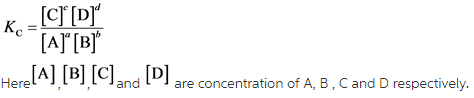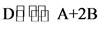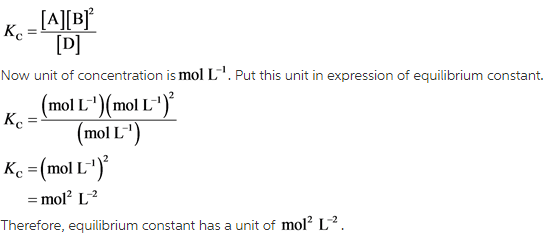# Calculate the value of the equilibrium constant for the reaction: D in equilibrium with A+2B A+2B

Calculate the value of the equilibrium constant for the reaction: D in equilibrium with A+2B A+2B in equilibrium with 2C Kc= 2.35 2C in equilibrium with D Kc= 0.182

Concepts and reason
The concept used to solve this problem is based on the equilibrium constant of a chemical reaction.
In a chemical reaction, the equilibrium constant is the value of reaction quotient when reaction attained equilibrium.

Fundamentals
For a reaction,The equilibrium constant,can be written as